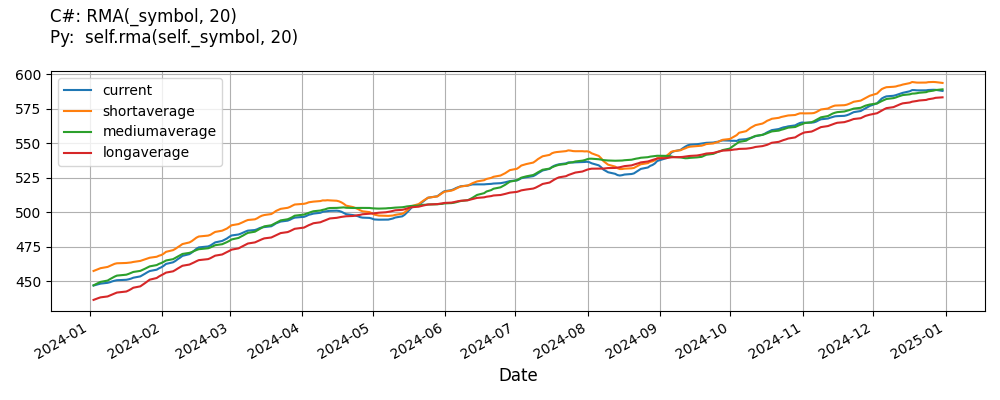# Supported Indicators

## Relative Moving Average

### Introduction

This indicator represents the relative moving average indicator (RMA). RMA = SMA(3 x Period) - SMA(2 x Period) + SMA(1 x Period) per formula: https://www.hybrid-solutions.com/plugins/client-vtl-plugins/free/rma.html

To view the implementation of this indicator, see the LEAN GitHub repository.

### Using RMA Indicator

To create an automatic indicators for RelativeMovingAverage, call the RMA helper method from the QCAlgorithm class. The RMA method creates a RelativeMovingAverage object, hooks it up for automatic updates, and returns it so you can used it in your algorithm. In most cases, you should call the helper method in the Initialize method.

public class RelativeMovingAverageAlgorithm : QCAlgorithm
{
private Symbol _symbol;
private RelativeMovingAverage _rma;

public override void Initialize()
{
_rma = RMA(_symbol, 20);
}

public override void OnData(Slice data)
{
{
// The current value of _rma is represented by itself (_rma)
// or _rma.Current.Value
Plot("RelativeMovingAverage", "rma", _rma);
// Plot all properties of rma
Plot("RelativeMovingAverage", "shortaverage", _rma.ShortAverage);
Plot("RelativeMovingAverage", "mediumaverage", _rma.MediumAverage);
Plot("RelativeMovingAverage", "longaverage", _rma.LongAverage);
}
}
}
class RelativeMovingAverageAlgorithm(QCAlgorithm):
def Initialize(self) -> None:
self.rma = self.RMA(self.symbol, 20)

def OnData(self, slice: Slice) -> None:
# The current value of self.rma is represented by self.rma.Current.Value
self.Plot("RelativeMovingAverage", "rma", self.rma.Current.Value)
# Plot all attributes of self.rma
self.Plot("RelativeMovingAverage", "shortaverage", self.rma.ShortAverage.Current.Value)
self.Plot("RelativeMovingAverage", "mediumaverage", self.rma.MediumAverage.Current.Value)
self.Plot("RelativeMovingAverage", "longaverage", self.rma.LongAverage.Current.Value)


The following reference table describes the RMA method:

### RMA()1/1

            RelativeMovingAverage QuantConnect.Algorithm.QCAlgorithm.RMA (
Symbol                           symbol,
Int32                            period,
*Nullable<Resolution>      resolution,
*Func<IBaseData, Decimal>  selector
)


Creates a new Relative Moving Average indicator for the symbol. The indicator will be automatically updated on the given resolution.

If you don't provide a resolution, it defaults to the security resolution. If you provide a resolution, it must be greater than or equal to the resolution of the security. For instance, if you subscribe to hourly data for a security, you should update its indicator with data that spans 1 hour or longer.

You can manually create a RelativeMovingAverage indicator, so it doesn't automatically update. Manual indicators let you update their values with any data you choose.

Updating your indicator manually enables you to control when the indicator is updated and what data you use to update it. To manually update the indicator, call the Update method with time/number pair, or an IndicatorDataPoint. The indicator will only be ready after you prime it with enough data.

public class RelativeMovingAverageAlgorithm : QCAlgorithm
{
private Symbol _symbol;
private RelativeMovingAverage _rma;

public override void Initialize()
{
_rma = new RelativeMovingAverage(20);
}

public override void OnData(Slice data)
{
if (data.Bars.TryGeValue(_symbol, out var bar))
{
_rma.Update(bar.EndTime, bar.Close);
}

{
// The current value of _rma is represented by itself (_rma)
// or _rma.Current.Value
Plot("RelativeMovingAverage", "rma", _rma);
// Plot all properties of rma
Plot("RelativeMovingAverage", "shortaverage", _rma.ShortAverage);
Plot("RelativeMovingAverage", "mediumaverage", _rma.MediumAverage);
Plot("RelativeMovingAverage", "longaverage", _rma.LongAverage);
}
}
}
class RelativeMovingAverageAlgorithm(QCAlgorithm):
def Initialize(self) -> None:
self.rma = RelativeMovingAverage(20)

def OnData(self, slice: Slice) -> None:
bar = slice.Bars.get(self.symbol)
if bar:
self.rma.Update(bar.EndTime, bar.Close)

# The current value of self.rma is represented by self.rma.Current.Value
self.Plot("RelativeMovingAverage", "rma", self.rma.Current.Value)
# Plot all attributes of self.rma
self.Plot("RelativeMovingAverage", "shortaverage", self.rma.ShortAverage.Current.Value)
self.Plot("RelativeMovingAverage", "mediumaverage", self.rma.MediumAverage.Current.Value)
self.Plot("RelativeMovingAverage", "longaverage", self.rma.LongAverage.Current.Value)


To register a manual indicator for automatic updates with the security data, call the RegisterIndicator method.

public class RelativeMovingAverageAlgorithm : QCAlgorithm
{
private Symbol _symbol;
private RelativeMovingAverage _rma;

public override void Initialize()
{
_rma = new RelativeMovingAverage(20);
RegisterIndicator(_symbol, _rma, Resolution.Daily);
}

public override void OnData(Slice data)
{
{
// The current value of _rma is represented by itself (_rma)
// or _rma.Current.Value
Plot("RelativeMovingAverage", "rma", _rma);
// Plot all properties of rma
Plot("RelativeMovingAverage", "shortaverage", _rma.ShortAverage);
Plot("RelativeMovingAverage", "mediumaverage", _rma.MediumAverage);
Plot("RelativeMovingAverage", "longaverage", _rma.LongAverage);
}
}
}
class RelativeMovingAverageAlgorithm(QCAlgorithm):
def Initialize(self) -> None:
self.rma = RelativeMovingAverage(20)
self.RegisterIndicator(self.symbol, self.rma, Resolution.Daily)

def OnData(self, slice: Slice) -> None:
# The current value of self.rma is represented by self.rma.Current.Value
self.Plot("RelativeMovingAverage", "rma", self.rma.Current.Value)
# Plot all attributes of self.rma
self.Plot("RelativeMovingAverage", "shortaverage", self.rma.ShortAverage.Current.Value)
self.Plot("RelativeMovingAverage", "mediumaverage", self.rma.MediumAverage.Current.Value)
self.Plot("RelativeMovingAverage", "longaverage", self.rma.LongAverage.Current.Value)


The following reference table describes the RelativeMovingAverage constructor:

### RelativeMovingAverage()1/2

            RelativeMovingAverage QuantConnect.Indicators.RelativeMovingAverage (
string  name,
int     period
)


Initializes a new instance of the RelativeMovingAverage class with the specified name and period.

### RelativeMovingAverage()2/2

            RelativeMovingAverage QuantConnect.Indicators.RelativeMovingAverage (
int  period
)


Initializes a new instance of the SimpleMovingAverage class with the default name and period.

### Visualization

The following image shows plot values of selected properties of RelativeMovingAverage using the plotly library.You can also see our Videos. You can also get in touch with us via Discord.